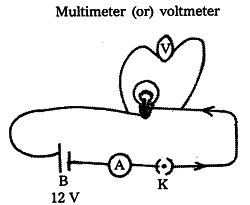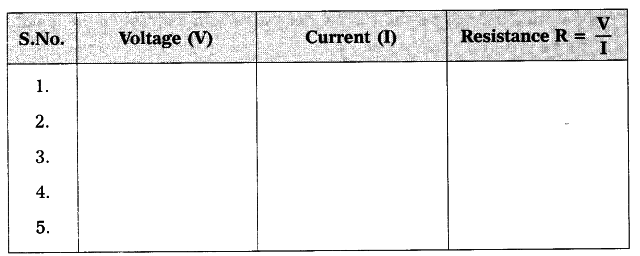# Take a battery and measure the potential difference

(a) Take a battery and measure the potential difference. Make a circuit and measure the potential difference when the battery is connected in the circuit. Is there any difference in potential difference of battery ?
(b) Measure the resistance of a bulb (filament) in open circuit with a multi-meter. Make a circuit with elements such as bulb, battery of 12 V and key in series. Close the key. Then again measure the resistance of the same bulb (filament) for every 30 seconds. Record the observations in a proper table. What can you conclude from the above results ?

(a) When the battery is connected in a circuit, the voltage slowly decreases due to consumption of it. So, there is difference in voltage before using and after connecting.
(b) After connecting battery (12 V), key in ammeter and bulb as shown in figure we measure current (I) using the ammeter and voltage using multimeter or voltmeter.
Note these values in the below table.
Measure the resistance of the same bulb for every 30 seconds.We conclude that the resistance is constant.